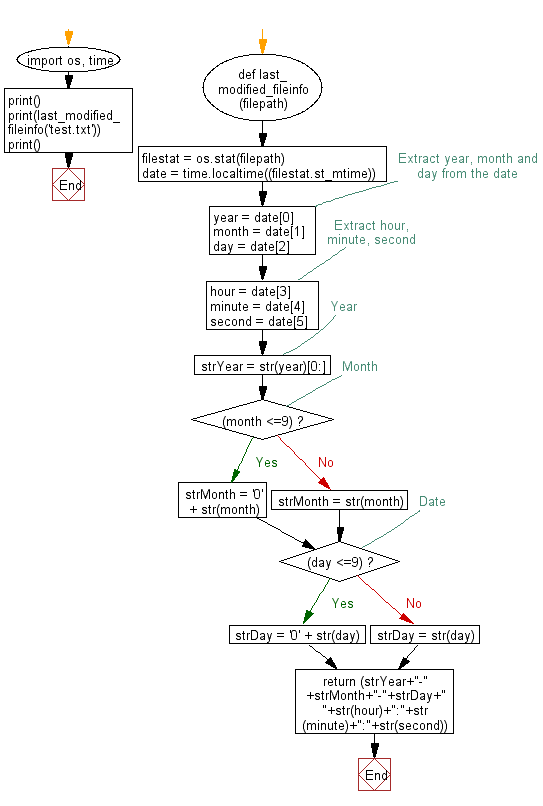## Python Datetime: Exercise-38 with Solution

Write a Python program to get the last modified information of a file.

Sample Solution:

Python Code:

``````import os, time
def last_modified_fileinfo(filepath):

filestat = os.stat(filepath)
date = time.localtime((filestat.st_mtime))

# Extract year, month and day from the date
year = date
month = date
day = date
# Extract hour, minute, second
hour = date
minute = date
second = date

# Year
strYear = str(year)[0:]

# Month
if (month <=9):
strMonth = '0' + str(month)
else:
strMonth = str(month)

# Date
if (day <=9):
strDay = '0' + str(day)
else:
strDay = str(day)

return (strYear+"-"+strMonth+"-"+strDay+" "+str(hour)+":"+str(minute)+":"+str(second))
print()
print(last_modified_fileinfo('test.txt'))
print()
```
```

Sample Output:

```2017-04-19 15:15:52
```

Flowchart:Python Code Editor: